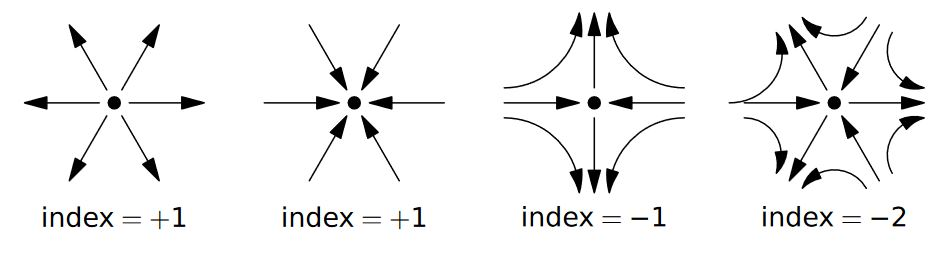# nLab Poincaré–Hopf theorem

Contents

### Context

#### Differential geometry

synthetic differential geometry

Introductions

from point-set topology to differentiable manifolds

Differentials

V-manifolds

smooth space

Tangency

The magic algebraic facts

Theorems

Axiomatics

cohesion

• (shape modality $\dashv$ flat modality $\dashv$ sharp modality)

$(\esh \dashv \flat \dashv \sharp )$

• dR-shape modality$\dashv$ dR-flat modality

$\esh_{dR} \dashv \flat_{dR}$

infinitesimal cohesion

tangent cohesion

differential cohesion

singular cohesion

$\array{ && id &\dashv& id \\ && \vee && \vee \\ &\stackrel{fermionic}{}& \rightrightarrows &\dashv& \rightsquigarrow & \stackrel{bosonic}{} \\ && \bot && \bot \\ &\stackrel{bosonic}{} & \rightsquigarrow &\dashv& \mathrm{R}\!\!\mathrm{h} & \stackrel{rheonomic}{} \\ && \vee && \vee \\ &\stackrel{reduced}{} & \Re &\dashv& \Im & \stackrel{infinitesimal}{} \\ && \bot && \bot \\ &\stackrel{infinitesimal}{}& \Im &\dashv& \& & \stackrel{\text{étale}}{} \\ && \vee && \vee \\ &\stackrel{cohesive}{}& \esh &\dashv& \flat & \stackrel{discrete}{} \\ && \bot && \bot \\ &\stackrel{discrete}{}& \flat &\dashv& \sharp & \stackrel{continuous}{} \\ && \vee && \vee \\ && \emptyset &\dashv& \ast }$

Models

Lie theory, ∞-Lie theory

differential equations, variational calculus

Chern-Weil theory, ∞-Chern-Weil theory

Cartan geometry (super, higher)

# Contents

## Idea

The Poincaré–Hopf theorem says that for any vector field $v \in \Gamma(T X)$ with a finite set of isolated vanishing points $\{x_i\}$ on an orientable compact differential manifold $X$, the sum over the $x_i \in X$ of the degrees of the vector in the vicinity of these points, regarded as cohomotopy classes

$v/{\vert v\vert}_{\vert x_i} \;\colon\; \partial D_{x_i} \longrightarrow S(T_{x_i} X)$

and called the Poincaré–Hopf index of $f$ at $x_i$

$ind_{x_i}(v) \;\coloneqq\; deg\big( v/{\vert v\vert}_{\vert {x_i} } \big)$

is given by the Euler characteristic, hence by the value of the Euler class on the tangent bundle:

$\chi(X) \coloneqq \chi[T X] \;=\; \underset{ {\text{isolated zero}} \atop { x_i } }{\sum} ind_{x_i}(v) \,.$In particular, the existence of a nowhere vanishing vector field (for which the above sum is empty) implies that the Euler characteristic vanishes.

The converse also holds: If the Euler characteristic of a closed manifold vanishes, then a nowhere vanishing vector field exists (e.g. MO:a/47553/58526).

Also: Given any connected closed manifold, the complement of any positive number of disjoint open balls carries a nowhere vanishing vector field (namely: choose any vector field with isolated zeros on the original manifold and then deform continuously to push all these zeros into inside the open balls). Smoothly scaling this vector field to zero towards the centers of these open balls gives a vector field on the original manifold which may be used in the PH-theorem.

Named after Henri Poincaré and Heinz Hopf.

Textbook accounts:

Review:

• Alex Wright, Kael Dixon, The Poincaré–Hopf theorem (pdf)

• Ariel Hafftka, Differential topology and the Poincaré–Hopf theorem (pdf)